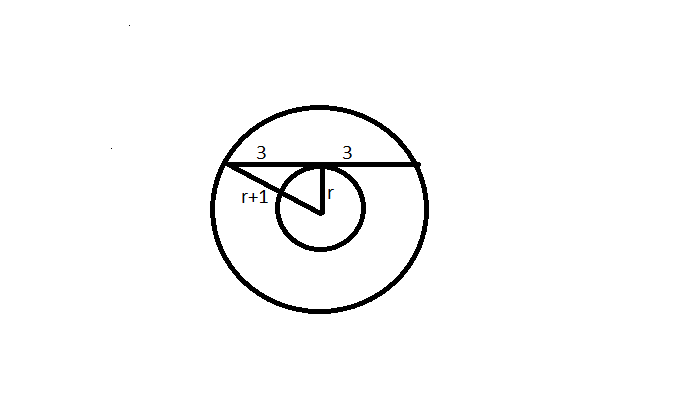Question 65

# Let C1 and C2 be concentric circles such that the diameter of C1 is 2cm longer than that of C2. If a chord of C1 has length 6cm and is a tangent to C2, then the diameter, in cm, of C1 is

Solution

Now we know that the perpendicular from the centre to a chord bisects the chord. Hence at the point of intersection of tangent, the chord will be divided into two parts of 3 cm each. As you can clearly see in the diagram, a right angled triangle is formed there.

Hence   $$\left(r+1\right)^2=r^2+9$$ or $$r^{2\ }+1+2r\ =\ r^2+9\ or\ 2r=8\ or\ r=4cm$$

Hence the radius of the larger circle is 5cm and diameter is 10cm.